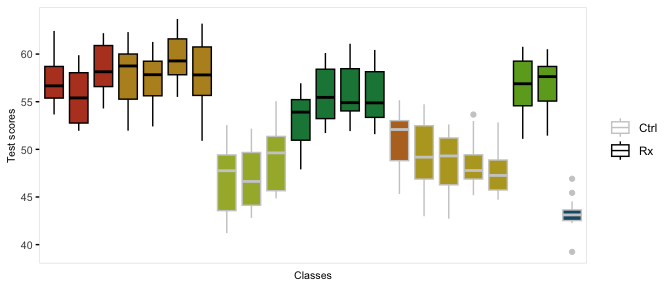# Clustered Data

The function genCluster generates multilevel or clustered data based on a previously generated data set that is one “level” up from the clustered data. For example, if there is a data set that contains the school level (considered here to be level 2), classrooms (level 1) can be generated. And then, students (now level 1) can be generated within classrooms (now level 2)

In the example here, we do in fact generate school-, class-, and student-level data. There are eight schools, four of which are randomized to receive an intervention. The number of classes per school varies, as does the number of students per class. (It is straightforward to generate fully balanced data by using constant values.) The outcome of interest is a test score, which is influenced by gender and the intervention. In addition, test scores vary by schools, and by classrooms, so the simulation provides random effects at each of these levels.

We start by defining the school level data:

gen.school <- defData(varname = "s0", dist = "normal", formula = 0, variance = 3,
id = "idSchool")
gen.school <- defData(gen.school, varname = "nClasses", dist = "noZeroPoisson", formula = 3)

set.seed(282721)

dtSchool <- genData(8, gen.school)
dtSchool <- trtAssign(dtSchool, n = 2)

dtSchool
##    idSchool         s0 nClasses trtGrp
## 1:        1  0.9732297        3      1
## 2:        2  3.5741932        4      1
## 3:        3  0.1121028        3      0
## 4:        4  0.5147236        4      1
## 5:        5  0.4594058        1      0
## 6:        6 -0.1287554        4      0
## 7:        7  2.4400170        2      1
## 8:        8 -1.2496060        1      0

The classroom level data are generated with a call to genCluster, and then school level data is added by a call to addColumns:

gen.class <- defDataAdd(varname = "c0", dist = "normal", formula = 0, variance = 2)
gen.class <- defDataAdd(gen.class, varname = "nStudents", dist = "noZeroPoisson",
formula = 20)

dtClass <- genCluster(dtSchool, "idSchool", numIndsVar = "nClasses", level1ID = "idClass")

head(dtClass, 10)
##     idSchool        s0 nClasses trtGrp idClass          c0 nStudents
##  1:        1 0.9732297        3      1       1  1.62726560        16
##  2:        1 0.9732297        3      1       2 -0.69640102        16
##  3:        1 0.9732297        3      1       3  1.53921338        13
##  4:        2 3.5741932        4      1       4 -1.58765603        30
##  5:        2 3.5741932        4      1       5 -2.35483046        26
##  6:        2 3.5741932        4      1       6  0.45956895        20
##  7:        2 3.5741932        4      1       7 -0.88401500        26
##  8:        3 0.1121028        3      0       8 -1.46100446        19
##  9:        3 0.1121028        3      0       9  0.07024057        19
## 10:        3 0.1121028        3      0      10  1.09465368        21

Finally, the student level data are added using the same process:

gen.student <- defDataAdd(varname = "Male", dist = "binary",
formula = 0.5)
gen.student <- defDataAdd(gen.student, varname = "age", dist = "uniform",
formula = "9.5; 10.5")
gen.student <- defDataAdd(gen.student, varname = "test", dist = "normal",
formula = "50 - 5*Male + s0 + c0 + 8 * trtGrp", variance = 2)
dtStudent <- genCluster(dtClass, cLevelVar = "idClass", numIndsVar = "nStudents",
level1ID = "idChild")

dtStudent <- addColumns(gen.student, dtStudent)

This is what the clustered data look like. Each classroom is represented by a box, and each school is represented by a color. The intervention group is highlighted by dark outlines:

## Warning: The guide argument in scale_*() cannot be FALSE. This was deprecated in
## ggplot2 3.3.4.
## This warning is displayed once every 8 hours.
## Call lifecycle::last_lifecycle_warnings() to see where this warning was
## generated.### Setting cluster sizes

It could be helpful to relax the assumption of exactly balanced cluster size when estimating statistical power using simulation. There is a “distribution” called clusterSize that facilitates these stochastic cluster sizes. As part of data definitions, you can specify the overall sample size N in the formula argument, and a dispersion parameter (in the variance field) Indicating a dispersion of 0 (the default) implies exact balance, and larger values of dispersion imply more variability in the cluster sizes.

Here are two examples with exact or close to exact balance:

d1 <- defData(varname = "clustSize", formula = 120, dist = "clusterSize")

genData(8, d1, id = "site")
##    site clustSize
## 1:    1        15
## 2:    2        15
## 3:    3        15
## 4:    4        15
## 5:    5        15
## 6:    6        15
## 7:    7        15
## 8:    8        15
genData(7, d1, id = "site")
##    site clustSize
## 1:    1        17
## 2:    2        17
## 3:    3        17
## 4:    4        17
## 5:    5        17
## 6:    6        18
## 7:    7        17

This is a second example with variability across sites:

d1 <- defData(varname = "clustSize", formula = 120,
variance = .1, dist = "clusterSize")

genData(8, d1, id = "site")
##    site clustSize
## 1:    1        22
## 2:    2        14
## 3:    3        14
## 4:    4        11
## 5:    5        12
## 6:    6        10
## 7:    7        18
## 8:    8        19